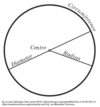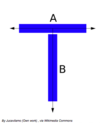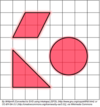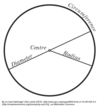# Mathematics & Measurements Flashcards Preview

## Ω Knowledge Rehab Ω > Mathematics & Measurements > Flashcards

Flashcards in Mathematics & Measurements Deck (35):
1

## If you have the time, Circumference

### Circumference is the perimeter of a circle.The circumference of a circle can be calculated using radius or diameter: C = 2 * π * r or C = π * d2

3

4

## Which French philosopher and mathematician is famous for his creation of the Cartesian coordinate system, still used today in algebra and geometry?

### René Descartes (1596-1650)You may remember the Cartesian coordinate system as a plot of X, Y on a two-dimensional axis.  This changed the way mathematicians were able to describe functions (correlational relationships).5

6

7

## Diameter

### Diameter is the distance from one side of a circle to the other measured in a straight line through the center.8

9

10

## normal distribution

### Bell-shaped, symmetric curve that represents data about many natural phenomena throughout the population11

12

## perpendicular

### Lines that form a 90º angle at their intersection.13

14

15

## Area

### Area is the measure of space on a surface. It is measured in units squared.Common area equations include: Circle: A = π r^2 Triangle: A = 1/2 b * h Rectangle: A = s1 * s2 Parallelogram: A = b * h16

17

18

19

20

21

## parallel

### Lines that are the same distance apart at every point along their length and never touch are called parallel.22

23

## What are the qualifications for a number to be considered prime?

24

### The distance from the center of a circle to its edge.25

26

27

28

29

30

31

32

33

34

35